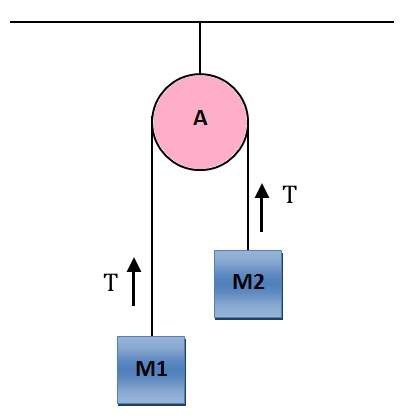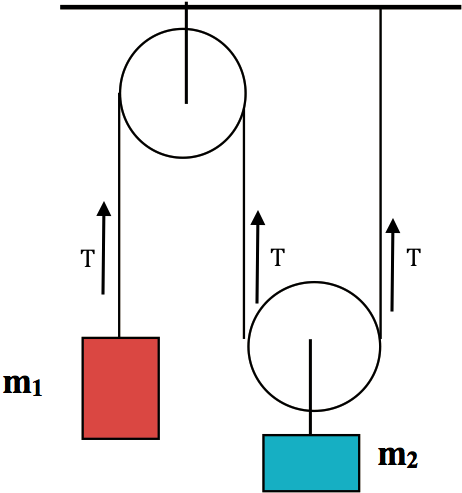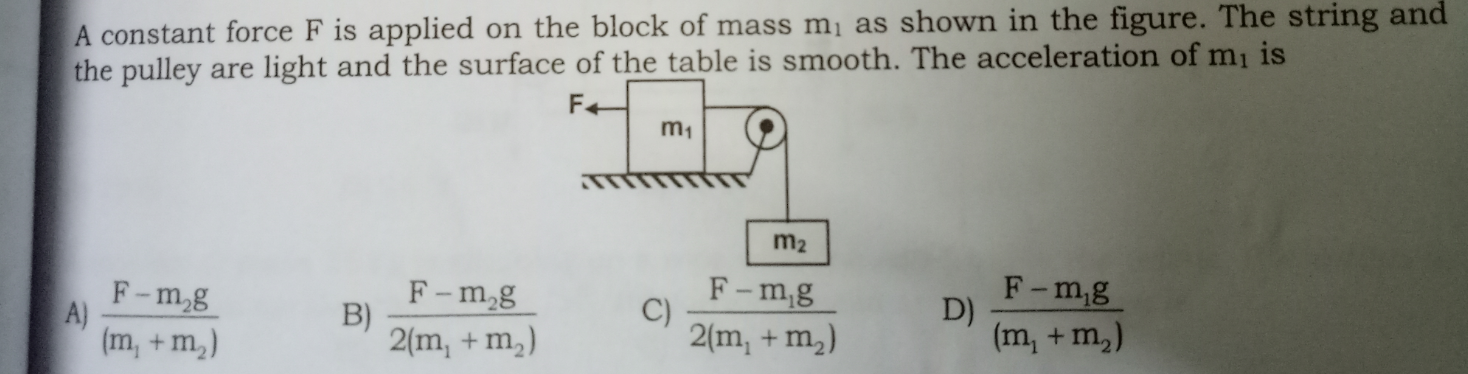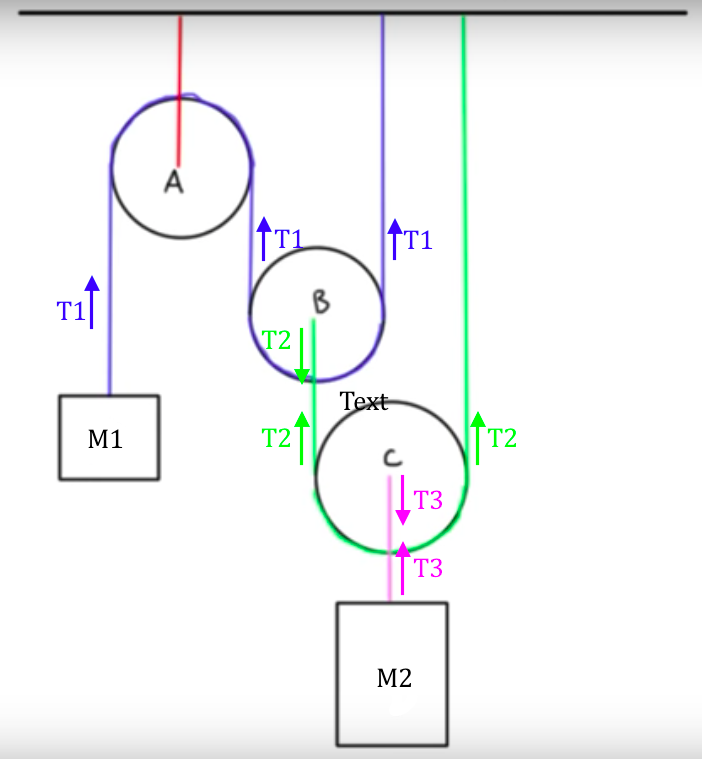D

#### Virtual work method

12 viewed last edited 3 years agospacegeek
0

I need a summary on the virtual work method used to find the relationship between accelerations of masses attached to a pulleyMahesh Godavarti
2

Method of virtual work is used to find the relationship between accelerations in various parts of a constrained system. Pulley-mass systems are usually constrained by pulleys being attached to an immovable surface and the masses attached to the system via strings. The virtual work method says that the work done by forces internal to the system (i.e. not external forces) is zero. Let's use this to find relative accelerations of various bodies in the system.In this the total virtual work done by the internal force T should be zero.

Therefore T X d1 + T X d2 = 0 => T X a1 + T X a2 = 0 (by differentiating T.d1 + T.d2 twice with respect to time, if you don't know what this means, don't worry about it for now). Important thing is to note that T X a1 + T X a2 = 0 => a1 = -a2. This makes obvious sense.

Now, let's try a slightly more complex system. Assume that the pulleys are masslessIn this we have T X a1 + 2T X a2 = 0 => a2 = -a1/2

I will post another answer soon with an even more complex example.Vivekanand Vellanki
1

The virtual work method says that: \sum_{k=1}^nT_k.a_k\ =\ 0 where Tk is the net internal force of object k and ak is the acceleration of object k. The product is the dot product.

This makes it simple to solve some questions by providing a way to compare the accelerations.

Lets apply this to the below:The net internal force on m1 is T and the net internal force on m2 is also T.

Virtual work method says

-Ta1 + Ta2 = 0; this implies a1 = a2.

I assumed a1 to be in the direction of F, hence the dot product of T and a1 is -Ta1.Mahesh Godavarti
1

Method of virtual work is used to find the relationship between accelerations in various parts of a constrained system. Pulley-mass systems are usually constrained by pulleys being attached to an immovable surface and the masses attached to the system via strings. The virtual work method says that the work done by forces internal to the system (i.e. not external forces) is zero. Let's use this to find relative accelerations of various bodies in the following system.If we consider C and M2 as one system then using the principle of virtual work we see that T3 X Ac - T3 X A2 = 0 => Ac = A2 where Ac is the acceleration of pulley C and A2 is the acceleration of Mass M2.

If we consider mass M1, pulleys A ad B and the ceiling as one system then, we get T1 X A1 + 2 T1 X Ab = 0 => Ab = -A1/2 where A1 is the acceleration of mass M1 and Ab is the acceleration of the pulley B.

If we assume all pulleys are massless then actual work done to move pulley B or pulley C is zero.

Therefore, considering pulley B as one system, the total work done by external forces (2T1 and T2) to move pulley B would be zero. Therefore, 2T1 X Ab - T2 X Ab = 0 => T2 = 2T1.

Similarly, considering pulley C as one system, the total work done by external forces (2T2 and T3) to move pulley C would be zero. Therefore, 2T2 X Ac - T3 X Ac = 0 => T3 = 2T2.

If we consider pulleys B, C and the ceiling as one system and look at only the internal forces in that system we get

T1 X Ab - T2 X Ab + T2 X Ac = 0 => Ab = Ac X T2 / (T2 - T1) => Ab = 2 Ac

If we consider the entire system, then

T1 X A1 + 2 T1 X Ab - T2 X Ab + 2 T2 X Ac + T3 X A2 - T3 X Ac = 0.

Since, we already know that T3 X A2 - T3 X Ac = 0, we get

T1 X A1 + 2 T1 X Ab - T2 X Ab + 2 T2 X Ac = 0 => T1 X A1 + 2 T2 X Ac = 0 => T1 X A1 + 4 T1 X Ac = 0 => Ac = -A1/4.

Therefore, A2 = -A1/4.